• +91 9971497814
• info@interviewmaterial.com

# RD Chapter 10- Congruent Triangles Ex-10.2 Interview Questions Answers

### Related Subjects

Question 1 :
In the figure, OA and OB are opposite rays:
(i) If x = 25°, what is the value of y?
(ii) If y = 35°, what is the value of x?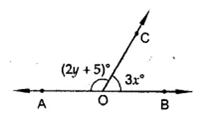(i) If x = 25°
∴ 3x = 3 x 25° = 75°
But ∠AOC + ∠BOC = 180° (Linear pair)
⇒ ∠AOC + 75° = 180°
⇒ ∠AOC = 180° – 75°
⇒ ∠AOC = 105°
∴ 2y + 5 = 105° ⇒ 2y= 105° – 5° = 100°
⇒ y =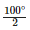= 50°
∴ If x = 25° then y = 50°
(ii) If y = 35°, then ∠AOC = 2y + 5
∴ 2y + 5 = 2 x 35° + 5 = 70° + 5 = 75°
But ∠AOC + ∠BOC = 180° (Linear pair)
⇒ 75° + ∠BOC = 180°
⇒ ∠BOC = 180°-75°= 105°
∴ 3x = 105° ⇒ x =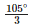= 35°
∴ x = 35°

Question 2 : In the figure, write all pairs of adjacent angles and all the linear pairs.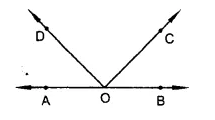In the given figure,
(i) ∠AOD, ∠COD; ∠BOC, ∠COD; ∠AOD, ∠BOD and ∠AOC, ∠BOC are the pairs of adjacent angles.
(ii) ∠AOD, ∠BOD and ∠AOC, ∠BOC are the pairs of linear pairs.

Question 3 : In the figure, find x, further find ∠BOC, ∠COD and ∠AOD.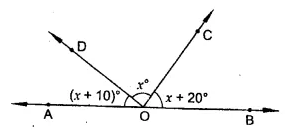In the figure,
AOB is a straight line
∴ ∠AOD + ∠DOB = 180° (Linear pair)
⇒ ∠AOD + ∠DOC + ∠COB = 180°
⇒ x+ 10° + x + x + 20 = 180°
⇒ 3x + 30° = 180°
⇒ 3x= 180° -30°= 150°
⇒ x =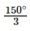= 50°
∴ x = 50°
Now ∠BOC =x + 20° = 50° + 20° = 70°
∠COD = x = 50°
and ∠AOD = x + 10° = 50° + 10° = 60°

Question 4 : In the figure, rays OA, OB, OC, OD and OE have the common end point O. Show that ∠AOB + ∠BOC + ∠COD + ∠DOE + ∠EOA = 360°.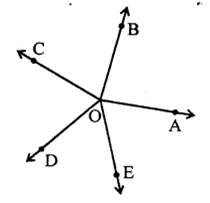Produce AO to F such that AOF is a straight line
Now ∠AOB + ∠BOF = 180° (Linear pair)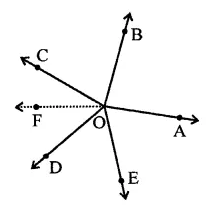⇒ ∠AOB + ∠BOC + ∠COF = 180° …(i)
Similarly, ∠AOE + ∠EOF = 180°
⇒ ∠AOE + ∠EOD + ∠DOF = 180° …(ii)
∠AOB + ∠BOC + ∠COF + ∠DOF + ∠EOD + ∠AOE = 180° + 180°
⇒ ∠AOB + ∠BOC + ∠COD + ∠DOE + ∠EOA = 360°
Hence proved.

Question 5 : In the figure, ∠AOC and ∠BOC form a linear pair. If a – 2b = 30°, find a and b.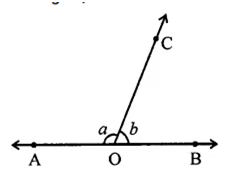∠AOC + ∠BOC = 180° (Linear pair)
⇒ a + 6 = 180° …(i)
and a – 2b = 30° …(ii)
Subtracting (ii) from (i), 3b = 150°
⇒ b == 50°
and a + 50° = 180°
⇒ a= 180°-50°= 130°
∴ a = 130°, b = 50°

Question 6 : How many pairs of adjacent angles are formed when two lines intersect in a point?

If two lines AB and CD intersect each other at a point O, then four pairs of linear pairs are formed i.e.
∠AOC, ∠BOC; ∠BOC, ∠BOD; ∠BOD, ∠AOD and ∠AOD, ∠AOC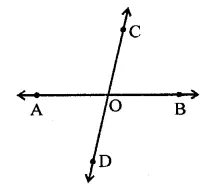Question 7 : How many pairs of adjacent angles, in all, can you name in the figure.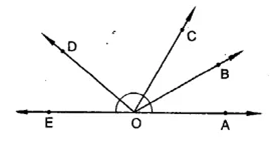In the given figure 10 pairs of adjacent angles are formed as given below:
∠AOB, ∠BOC; ∠AOB, ∠BOD; ∠AOC, ∠COD; ∠AOD, ∠BOE; ∠AOB, ∠BOE; ∠AOC, ∠COF; ∠BOC, ∠COD; ∠BOC, ∠COE; ∠COD, ∠DOE and ∠BOD, ∠DOE

Question 8 : In the figure, determine the value of x.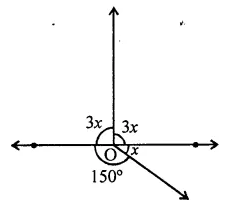In the figure
∠AOC + ∠COB + ∠BOD +∠AOD = 360 (angles at a point )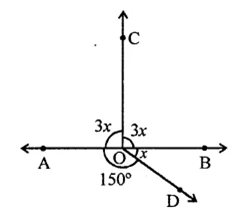⇒ 3x + 3x + x + 150° = 360°
⇒ 7x – 360° – 150° = 210°
⇒ x =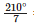= 30°
∴ x = 30°

Question 9 : In the figure, AOC is a line, find x.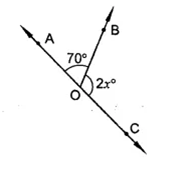In the figure,
∠AOB + ∠BOC = 180° (Linear pair)
⇒ 70° + 2x = 180°
⇒ 2x = 180° – 70°
⇒ 2x = 110°⇒x =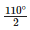= 55°
∴ x = 55°

Question 10 : In the figure, POS is a line, find x.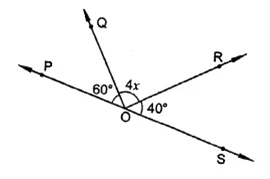In the figure, POS is a line
∴ ∠POQ + ∠QOS = 180° (Linear pair)
⇒ ∠POQ + ∠QOR + ∠ROS = 180°
⇒ 60° + 4x + 40° = 180°
⇒ 4x + 100° – 180°
⇒ 4x = 180° – 100° = 80°
⇒ x =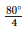=20°
∴ x = 20°

Todays Deals### RD Chapter 10- Congruent Triangles Ex-10.2 Contributorskrishan

Name:
Email:

# Latest News# 9000 interview questions in different categories